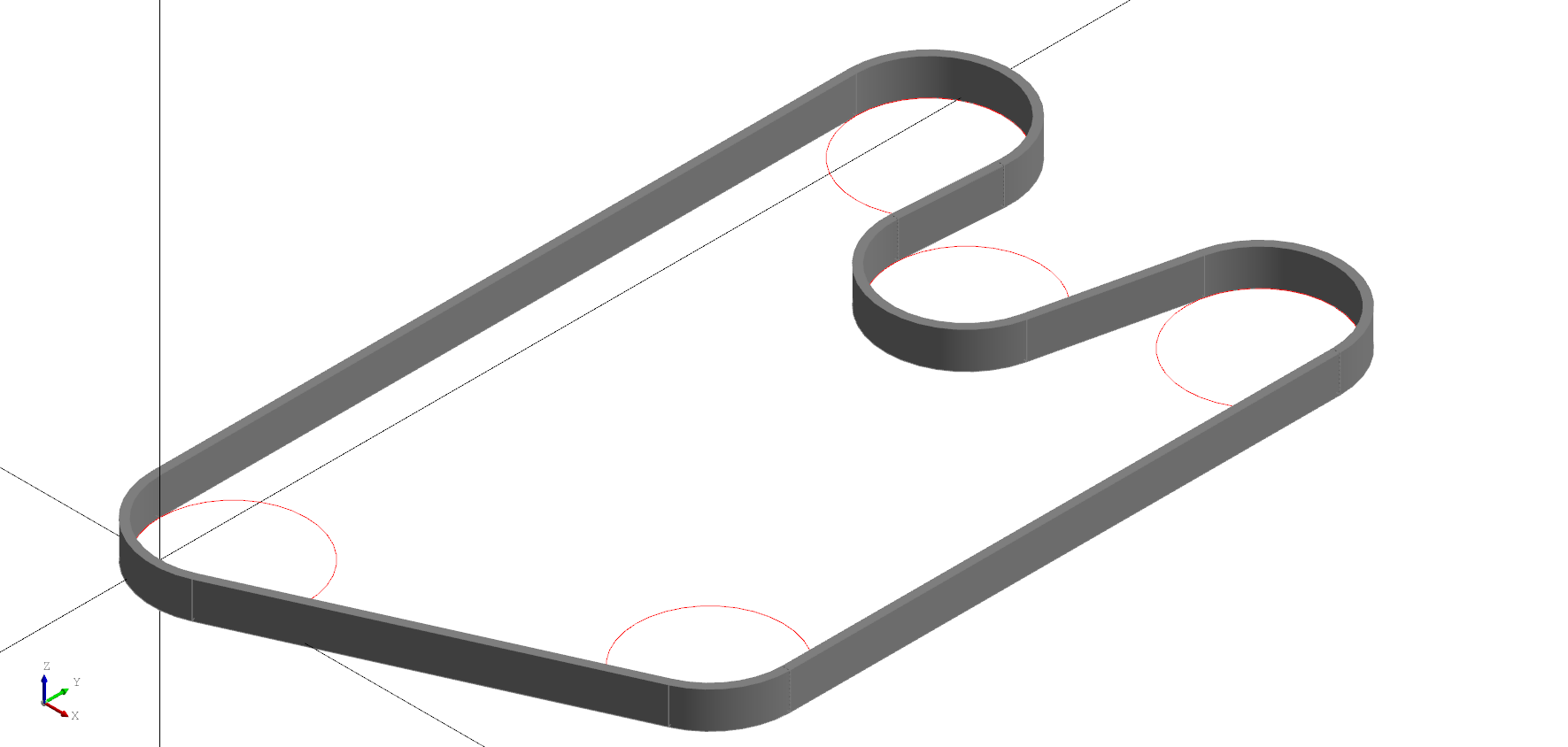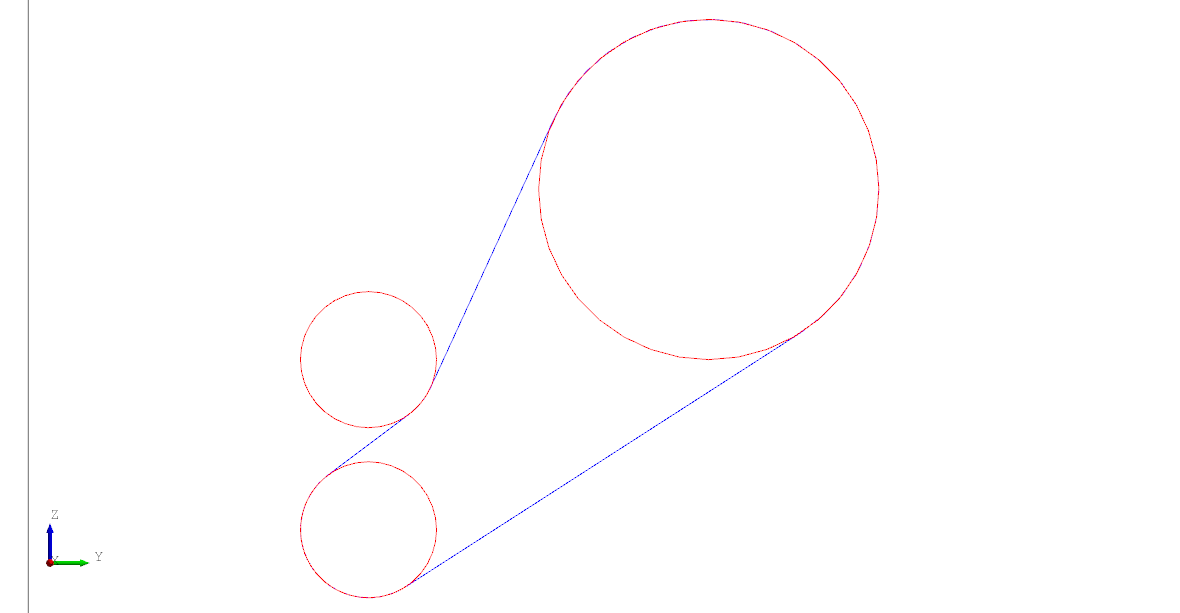### Circuit

The `Circuit` node is used create a wire following the path around a set of circles. Typically this is used for creating belts or chains around a set of gears or pulleys.

Specify the `circles` attribute which takes a list of circles to build the circuit from. Circles can take the form of a tuple in the format of `(radius, position)` or be an instance of a `Circle` node. All circles must be in a single plane. An `offset` value can be provided to add space around positive or negative turns as shown below. The offset value only applies to circles with the same side, eg a negative offset only is added to internal circles (those that also have a negative radius).

Note: A circle can be given a negative radius to indicate the path should go around the "inside". The `reverse=True` parameter (or a boolean in the tuple) will tell the solver to reverse the arc direction.

``````# Created in DeclaraCAD

enamldef Assembly(Part):
Axis:
pass

Include:
objects = belt.circles

Extrude:
vector = (0, 0, 1)
color = '#333'
Loft:
Circuit: belt:
offset = 0.2
circles = [
(2,  (1, 1)),
(2,   (10, 5)),
(2,   (10, 20)),
(-2,   (5, 17)),
(2,   (1, 20)),
]
Circuit:
offset = -0.2
circles = belt.circles
``````An example showing using `Circle` directly and specifying the `reverse` parameter. Alternatively you can set `reverse = True` on the `Circuit` instead of each circle.

``````# Created in DeclaraCAD

enamldef Assembly(Part):
Axis:
pass
Include:
objects = idler.circles
Circuit: idler:
color = 'blue'
circles = [
Circle(radius=2, reverse=True, position=(0, 10, 10), direction=(1, 0, 0)),
Circle(radius=-2, reverse=True, position=(0, 10, 15), direction=(1, 0, 0)),
Circle(radius=5, reverse=True, position=(0, 20, 20), direction=(1, 0, 0)),
]
``````Note: If only two circles are given it will use the `position` and `direction` of the first circle to define the "normal" plane.